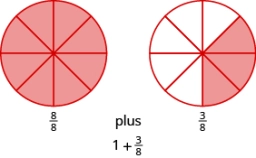# Solve 24

Solve the following equation, answer as a reduced, mixed number. Then place the correct number in the box provided.

4(2x -3) + 8(x - 4) = 2(2x + 6)

x =  4 23 = 14/3

### Step-by-step explanation:Did you find an error or inaccuracy? Feel free to write us. Thank you!

Tips for related online calculators
Need help calculating sum, simplifying, or multiplying fractions? Try our fraction calculator.
Need help with mixed numbers? Try our mixed-number calculator.
Do you have a linear equation or system of equations and looking for its solution? Or do you have a quadratic equation?